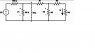# Basic Circuit Problem: Find Req, v1, v2, i4

• Engineering
• EngnrMatt
In summary, the equivalent resistance of the circuit at the dashed line is 139.612 ohms. The voltage at v1 is 35.58 V, the voltage at v2 is 29.867 V, and the current through i4 is 0.103 A. The steps taken to find the equivalent resistance are combining R3 and R4, then combining that with R2, then adding R1, then combining it with the parallel resistor, and finally adding the last resistor in parallel. However, there may be an alternative interpretation of the question that is not clear.

## Homework Statement

In the circuit below, VS = 65 V, R1 = 64 Ω, R2 = 190 Ω, R3 = 90 Ω, R4 = 290 Ω.
Find:

(a) The equivalent resistance (Req) at the dashed line
(b) v1
(c) v2
(d) i4

## The Attempt at a Solution

I found the equivalent resistance of the circuit (139.612 $\Omega$) by collapsing the circuit step-by-step, but that didn't seem to work. Apparently this line is referring to something else, and I'm not sure what to do.

#### Attachments

•3-4.png
6.6 KB · Views: 375
Can you show the steps you took to find the equivalent resistance? I'm not seeing the same result.

First I combine R3 and R4 (290+90) which gives 380. Then I combine that with its parallel R2 (190) which gives 1/[(1/380)+(1/190)] = 126.66, then I add that to R1 (64) which gives 190.66, then combine it with its parallel (50), giving 39.612, which I then add to the last resistor, 100, to get 139.612 ohms. If I am making a mistake, I'd like to know where.

EngnrMatt said:
First I combine R3 and R4 (290+90) which gives 380. Then I combine that with its parallel R2 (190) which gives 1/[(1/380)+(1/190)] = 126.66, then I add that to R1 (64) which gives 190.66, then combine it with its parallel (50), giving 39.612, which I then add to the last resistor, 100, to get 139.612 ohms. If I am making a mistake, I'd like to know where.

Ah. That last resistor, 100 Ω, is also in parallel, not in series; You're want to look at the circuit from the point of view of where the dotted line is, not from where VS is connected.

Okay, well I'm not sure how to handle it at that point then. I did everything the same as before, only with 100 in parallel, but that didn't seem to work, so I'm all out of ideas.

EngnrMatt said:
Okay, well I'm not sure how to handle it at that point then. I did everything the same as before, only with 100 in parallel, but that didn't seem to work, so I'm all out of ideas.
What value did you get?

28.373 ohms

EngnrMatt said:
28.373 ohms

That's what I'm seeing, too. So...

It's just possible that they want the equivalent resistance for the network to the left of the dotted line only. This would be a common step that one might do if one wanted to replace that piece of the circuit with a Thevenin equivalent for the source and network (you may or may not have learned about Thevenin equivalents yet).

Definitely not, considering this is the homework for just day 3 of circuits 1.

EngnrMatt said:
Definitely not, considering this is the homework for just day 3 of circuits 1.

Well, I don't know what else to suggest. That's the only likely alternative interpretation of the question that springs to mind.

•1 person